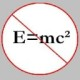# The Unapologetic Mathematician

## Subrepresentations and Quotient Representations

Today we consider subobjects and quotient objects in the category of representations of an algebra$A$. Since the objects are representations we call these “subrepresentations” and “quotient representations”.

As in any category, a subobject$\sigma$ (on the vector space$W$) of a representation$\rho$ (on the vector space$V$) is a monomorphism$f:\sigma\rightarrow\rho$. This natural transformation is specified by a single linear map$f:W\rightarrow V$. It’s straightforward to show that if$f$ is to be left-cancellable as an intertwinor, it must be left-cancellable as a linear map. That is, it must be an injective linear transformation from$W$ to$V$.

Thus we can identify$f$ with its image subspace$f(W)\subseteq V$. Even better, the naturality condition means that we can identify the$\sigma$ action of$A$ on$W$ with the restriction of$\rho$ to this subspace. The result is that we can define a subrepresentation of$\rho:A\rightarrow\mathrm{End}(V)$ as a subspace of$V$ so that$\rho(a)$ actually sends$W$ into itself for every$a\in A$. That is, it’s a subspace which is fixed by the action$\rho$.

If$\sigma$ is a subrepresentation of$\rho$, then we can put the structure of a representation on the quotient space$V/W$. Indeed, note that any vector in the quotient space is the coset$v+W$ of a vector$v\in V$. We define the quotient action using the action on$V$:$\left[\left[\rho/\sigma\right](a)\right](v+W)=\left[\rho(a)\right](v)+W$. But what if$v'=v+w$ is another representative of the same coset? Then we calculate:\displaystyle\begin{aligned}\left[\left[\rho/\sigma\right](a)\right](v'+W)=\left[\rho(a)\right](v')+W\\=\left[\rho(a)\right](v+w)+W\\=\left[\rho(a)\right](v)+\left[\rho(a)\right](w)+W\\=\left[\rho(a)\right](v)+W\\=\left[\left[\rho/\sigma\right](a)\right](v+W)\end{aligned}

because$\rho(a)$ sends the subspace$W$ back to itself.

December 5, 2008 - Posted by | Algebra, Representation Theory

1. Too much metaphor, too little concrete.

NSComment by notedscholar | December 5, 2008 | Reply

2. Not interested, NS.Comment by John Armstrong | December 5, 2008 | Reply

3. Not to prolong a discussion the host has no interest in pursuing, but since this is the second time NS has charged that John is indulging in metaphor, it should at least be said that all the terms John is using are completely standard mathematical jargon. So the charge is wildly off base.Comment by Todd Trimble | December 7, 2008 | Reply

4. Oh, NS won’t be happy unless everything is expressed in terms of a bunch of rocks. He’s another gadfly, although I will give him credit for having his own weblog to expound his own ideas, unlike the last we had around here.Comment by John Armstrong | December 7, 2008 | Reply

5. […] us two subspaces: the kernel and the image . And it turns out that each of these is actually a subrepresentation. Showing this isn’t difficult. A subrepresentation is just a subspace that gets sent to […]

Pingback by Kernels and Images of Intertwiners « The Unapologetic Mathematician | December 12, 2008 | Reply

6. […] the form to itself. This defines a one-dimensional subspace fixed by the representation — a subrepresentation defined […]

Pingback by Do Short Exact Sequences of Representations Split? « The Unapologetic Mathematician | December 17, 2008 | Reply

7. […] interesting properties. Each one, given a representation of the symmetric group, will give us a subrepresentation of that […]

Pingback by The Symmetrizer and Antisymmetrizer « The Unapologetic Mathematician | December 19, 2008 | Reply